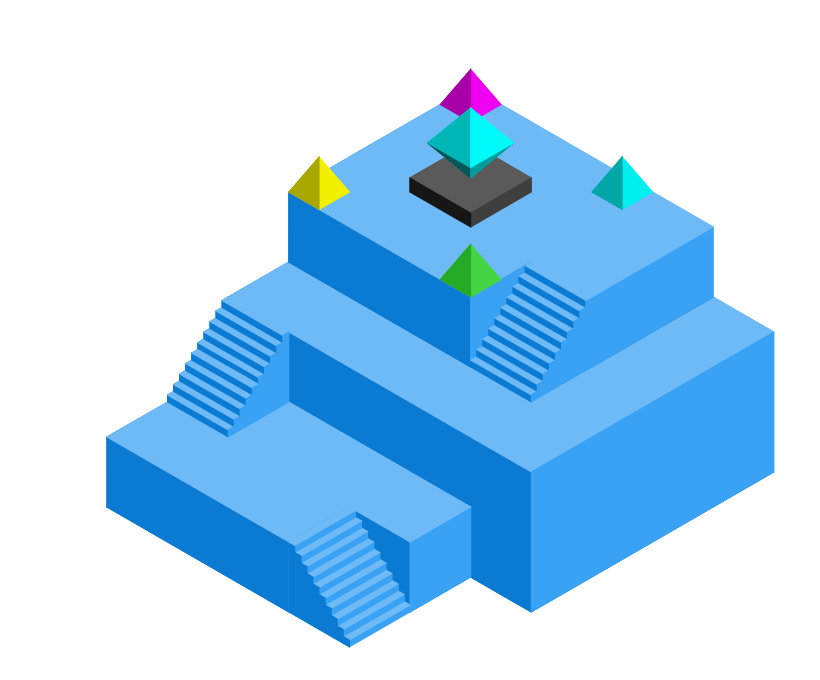## Isometric Design Android LibraryIsometric drawing is way of presenting designs/drawings in three dimensions. In order for a design to appear three dimensional, a 30 degree angle is applied to its sides. The cube opposite, has been drawn in isometric projection.## What is purpose of isometric design Android?

1. You can use it for game development where you want to create 3D drawing.
2. Blueprint of any house or 3D model.
3. Small application for learner kids and many more what your thinking can do.

Lets come with implementing the isometric design Android library.

### Using JCenter

`compile 'io.fabianterhorst:Isometric:0.0.6'`

### Available Shapes

#### Cylinder#### Knot#### Octahedron#### Prism#### Pyramid#### Stairs### Translate

Traslate is translating an point, path or shape to the given x, y and z distance. Translate is returning a new point, path or shape.

```Prism prism = new Prism(new Point(0, 0, 0));
isometricView.add(prism.translate(0, 0, 1.1), new Color(33, 150, 243));```### Scale

Scale is scaling an point, path or shape with the given x, y and z scaling factors. Scale is returning a new point, path or shape.

```Color blue = new Color(50, 60, 160);
Color red = new Color(160, 60, 50);
Prism cube = new Prism(Point.ORIGIN);
.scale(Point.ORIGIN, 3.0, 3.0, 0.5)
.translate(0, 0, 0.6), blue);```### RotateZ

RotateZ is rotating an point, path or shape with the given angle in radians on the xy-plane (where an angle of 0 runs along the position x-axis). RotateZ is returning a new point, path or shape.

```Color blue = new Color(50, 60, 160);
Color red = new Color(160, 60, 50);
Prism cube = new Prism(Point.ORIGIN, 3, 3, 1);
/* (1.5, 1.5) is the center of the prism */
.rotateZ(new Point(1.5, 1.5, 0), Math.PI / 12)
.translate(0, 0, 1.1), blue);```### Shapes from Paths

The method `Shape.extrude` allows you to create a 3D model by popping out a 2D path along the z-axis.

```Color blue = new Color(50, 60, 160);
Color red = new Color(160, 60, 50);
isometricView.add(new Prism(Point.ORIGIN, 3, 3, 1), blue);
new Point(1, 1, 1),
new Point(2, 1, 1),
new Point(2, 3, 1)
}), 0.3), red);```### Drawing multiple Shapes

There are 3 basic components: points, paths and shapes. A shape needs an origin point and 3 measurements for the x, y and z axes. The default Prism constructor is setting all measurements to 1.

```isometricView.add(new Prism(new Point(0, 0, 0)), new Color(33, 150, 243));
isometricView.add(new Prism(new Point(-1, 1, 0), 1, 2, 1), new Color(33, 150, 243));
isometricView.add(new Prism(new Point(1, -1, 0), 2, 1, 1), new Color(33, 150, 243));```### Drawing multiple Paths

Paths are two dimensional. You can draw and color paths the same as shapes.

```isometricView.add(new Prism(Point.ORIGIN, 3, 3, 1), new Color(50, 60, 160));
new Point(1, 1, 1),
new Point(2, 1, 1),
new Point(2, 2, 1),
new Point(1, 2, 1)
}), new Color(50, 160, 60));```### The grid

Here you can see how the grid looks like. The blue grid is the xy-plane. The z-line is the z-axis.### Supports complex structuresHave you tried this library ever? If no then use this cool library.#### By Tell Me How

It is a technology blog and admin has excellent experience in programming from 5+ year. You can contact us at ceo.tellmehow@gmail.com

#### Most recommended Android Auto head unit stereo cars